Jekyll2022-12-31T20:45:08+00:00https://averylaird.com/feed.xmlaverylaird.comA place for my research news.Avery Lairdalaird@cs.toronto.eduPico Now Public2018-05-26T00:00:00+00:002018-05-26T00:00:00+00:00https://averylaird.com/programming/piece-table/pico/2018/05/26/publish-picoIn my post last week, I mentioned having a test “toy” version. It is dubbed “pico,” and is now hosted on a public github repo for anyone to look at, tinker with, or improve.

Pico is in the “barely works” phase, and only supports insertions for now. I will be working on this off and on over the next few weeks/months. Check it out if you’d like to contribute, or just take a look at the progress.

]]>
Avery Lairdalaird@cs.toronto.edu
Piece Tables, Splay Trees, and “Trables” (Oh My!)2018-05-10T00:00:00+00:002018-05-10T00:00:00+00:00https://averylaird.com/programming/piece-table/2018/05/10/insertions-piece-tableThis was a lot harder that I thought it would be (and if you’re not sure what “it” is, check out my last post). First, I tried using a red-black tree to store the pieces, similar to the method proposed by Joaquin Cuenca Abela. He has already written a very impressive C++ implementation. The caveat with red-black trees is that they have a lot of cases to deal with, and (in most situations) perform only slightly better than some alternatives. And, as it was once wisely said:

“...premature optimization is the root of all evil.”

Donald Knuth

When thinking about balanced binary trees, there are always three main structures to consider: AVL trees, splay trees, and normal (unbalanced) BST trees. It may seem like a contradiction to include unbalanced BSTs in a list of balanced trees; however, UBSTs can remained balanced as long as the keys being inserted are sufficiently unsorted. A text editor doesn’t usually meet this requirement.

AVL Trees usually perform very well, almost as well as red-black trees. If you’re unsure about the nature of the data being stored in the tree, the AVL tree works nicely. We can work a bit smarter though – there’s a simpler and faster solution to consider: Splay trees. This structure redistributes the nodes of a tree similar to AVL trees. The main difference is that we do not aim to balance the tree at all – we keep shifting around (“splaying”) nodes until the most recently inserted node is the root of the tree. And as I’ll explain below, we can “encode” the positions of pieces in the structure of the tree, eliminating the need to keep track of indices.

At first glance, it might seem that splay trees should not perform well at all, perhaps even worse than the UBST, because it is not particularly balanced. Surprisingly, the amortized time complexity of operations on a splay tree is $$O(logN)$$. This is because splay trees rely on the assumption that recently accessed data is the most likely to be required soon in the future. This is generally true, but especially relevant in the case of text editors. Most of the time, we are working on only a small section of the document.

Parts of Atom were recently rewritten to use a splay tree, with apparently pretty good results. I know that splay trees will perform very well with the common use case – I’m unsure how they will fare with large buffers and multiple cursors/concurrent editors. There’s only one way to find out.

“One good test is worth a thousand expert opinions.”

Wernher Von Braun

## Operations

When designing the buffer interface, two basic functions must be considered:

\begin{align} \mathrm{insert}(index, string) \\ \mathrm{delete}(span) \end{align}

A $$span$$ is an ordered set of two indices, $$(i_s, i_e)$$ which represents all characters within that range of indices (inclusive). In this post, I will handle only the insertion operation, and address deletion in a later post.

The $$index$$ is known to us through the cursor position, and the $$string$$ is given by user input along with $$span$$. All other values must be handled internally. We are storing the information about edits in a piece table, abstractly; but actually, since we keep each piece in a tree, it’s more of a “piece tree” (or a “trable?”). There are some conditions that we must place on this tree, mainly that an inorder traversal starting from the root of the tree results in a proper evaluation of the buffer. Because a node is equivalent to a piece, and a tree is equivalent to a table, I will use these terms interchangeably (depending on what is most appropriate in the context).

Although we can properly evaluate the entire table by starting at the root, and any section of the buffer by traversing a child node and its subtrees, we do not know where the section belongs in the buffer. As I’ll explain, there’s no way to know a node’s position in the buffer without any information about the rest of the tree. This is because we will use a very clever suggestion from Joaquin Abela, which allows us to store nodes based on their relative index — their index relative to other pieces in the table.

To implement this method, there are two changes we need to make to the typical splay tree: how we insert nodes, and how we store data. The procedure for inserting nodes is affected by the key we choose to sort the data. Intuitively, this should be something related to the position of a piece within the document. At first, we might consider using the desired insertion index, $$i_d$$.

The issue with using $$i_d$$ is that it must change for any insertion at index $$i \leq i_d$$. After such an insertion, we would have to update every node after $$i$$. In the case of several insertions at the beginning of an existing buffer, this cases a $$O(n)$$ time complexity for each insertion — no better than an array.

Joaquin Abela suggests a solution to this problem: store the offset information in the nodes themselves. He does this by storing subtree sizes, rather than indices:

        Explicit Indexing                         Relative Indexing

n1                                       n1
index:  14                             size_right: 1
length: 10                             size_left: 14
/         \           =>                length:    10
n2                   n3                       /             \
index:   0           index: 23           n2                        n3
length: 14           length: 1      size_right: 0              size_right: 0
/          \         /         \     size_left:  0              size_left:  0
A            B       C           D    length:    14              length:     1
/             \            /             \
A               B          C               D


It’s worth spending a bit of time talking about the example above, because there are two very important differences to consider. Firstly, note that n2 has a index of 0 and a length of 14, while n1 picks up at index 14. This is because size is 1-indexed (I do not consider strings of length 0 to have meaning) whereas index is 0-indexed. So, n2 actually spans indices 0 -> 13. I will also define an insertion at index $$i$$ to have the following behaviour:

0123456789ABCDEFGH
This is a sentence
^       insert 'i' @ index D
This is a senitence


Such that the index $$i$$ refers to the desired final position.

Secondly, and most importantly, note how the structure of the tree does not change in either case. This illustrates how the structure of the tree itself stores the correct order of the pieces relative to each other, and implies that any resources spent storing and updating the index is a waste. Unlike indices, this order is also constant even after splaying — once the node is inserted properly, everything works fine.

Finally, we need to consider how an inserted piece may require the “split” of an existing piece. This involves devising a test to detect when a split should occur, and performing the split such that all properties of a piece table and a splay tree are preserved (I will not give a particularly in-depth explanation of splay trees here, but the Wikipedia page is a good place to start).

### Insertions

To make an insertion, we will still need to consider the desired index, but we can forget it afterwards. I will go through an example below. We will use the same example as above, assuming the existing buffer was inserted as a single piece.

(1) Original Tree        (2) Insert 'i' @ index 13

size_right: 0            size_right: 0      Because:
size_left:  0            size_left:  0      offset <index 13 < offset+length
length:    18     -->    length:    18      we must split!
text:     0x0            text:     0x0

(2.1) View of subtree during split
/
size_right: 0
size_left: 13
length:     1
text:     0x1
/
size_right: 0
size_left:  0
length:    13
text:     0x2

(2.2) Join split subtree with parent node

size_right: 0
size_left:  0   <-- must update size_left
length:    18   <-- must update length
text:     0x0   <-- must update text pointer
/
size_right: 0
size_left: 13
length:     1
text:     0x1
/
size_right: 0
size_left:  0
length:    13
text:     0x2

(2.3) Update parent node

size_right: 0
size_left: 19  <-- 13+1+5
length:     5  <-- 18-13
text:     0x3  <-- explained below
/
size_right: 0
size_left: 13
length:     1
text:     0x1
/
size_right: 0
size_left:  0
length:    13
text:     0x2


Of course, there are actually (up to) 3 different ways to perform such a split. I choose to form a linked list on whatever side the node is supposed to be inserted, because we know there aren’t any children there. The other thing to consider is how memory is managed throughout. If we examine just the memory addresses mentioned above, we might see something like this:

(1)   0x0: This is a sentence

(2.1) 0x0: This is a sentence
0x1: i

(2.2) 0x0: This is a sentence
0x1: i
0x2: This is a sen

(2.3) 0x0: This is a sentence <-- here we can free(0x0)
0x1: i
0x2: This is a sen
0x3: tence


This method can be done without copying if we also store a start value in the node. We still need to allocate memory for the newly inserted character, but we would never have to change a pointer to memory once it is allocated. We can just increment the start value to be whatever the length of the first node in the split is. For example, the following memory contents and pointer/start pairs:

0x0 This is a sentence
0x1 i

0x0, start = 0 , length = 13
0x1, start = 1 , length = 1
0x0, start = 13, length = 5


The start value would be relative to the text pointer. We can get the section of text we want by reading from text+start by sizeof(text_type)*length bytes (the data we’re storing may or may not be a char).

Finally, if we do an inorder traversal of the example tree, we get:

This is a senitence
|-----------|||---|
piece1    |  |
piece2----|  |
piece3-------|


An insertion without a split would form a subtree of a single node, and perform the same join operation. However, only the size_left of the parent node (in this case) would be changed — the length and start values would not change. Actually, if we follow the process described above, a split will always join of the left side of a parent, because the desired insertion index is less than the span of that piece.

## Code

First we will need a piece, which is just a collection of three values:

I’m assuming we’re storing chars here, but we can replace this with a different type later if we have to. We will be storing these pieces in a tree structure:

In terms of types, that’s about it. I won’t post a bunch of code here, it’s all available on github. However, I will talk about the functions involved in insertions, and how I handle that process.

First, we follow the typical BST insertion algorithm. However, before performing the insertion, we perform a split test. If a split must be performed, we do it, otherwise, just insert the node normally. We record the address of the newly inserted node, and pass it to the splay function.

The splay function takes the address of the new node, and splays it up the tree until it is the root of the tree. That’s it! Sounded pretty easy to me the first time I tried to write it. And, maybe if I had better coding chops, it would’ve been. However, after a while, I did manage to get a testable base implementation.

## Testing

It’s hard to do useful, comprehensive tests in this case, since there are so many situations which may occur. However, I did some very rudimentary testing and benchmarking of the insert operation, with interesting results.

First, I inserted 1,000,000 characters into a piece table, which is equivalent to a dense document with 30,000 pages,1 giving the following graph: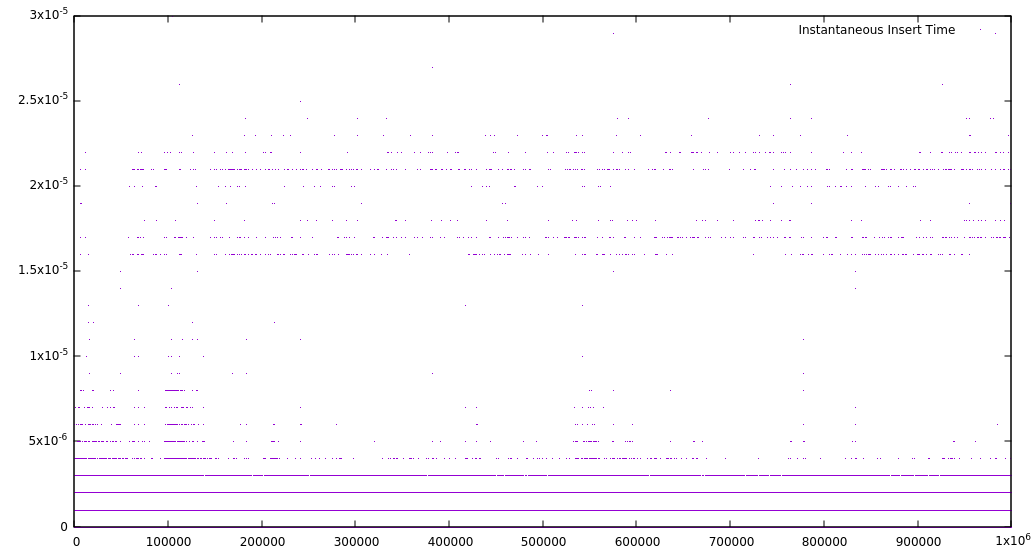This is certainly a strange looking graph indeed, and that is mostly related to the nature of a splay tree. My guess is that the straight lines represent operations close to each other, and therefore very fast. The much slower insertion times represent operations very far from previous ones, which then become fast due to the splay operation. This is not bad for a worst case, but not particularly impressive either. Things get interesting if we take a look at a different quantity.

Inserting characters randomly doesn’t give a fair representation of the common use case for most editors. To get a more balanced perspective, I also recorded the average time for each insertion into a table of a certain size. I kept a running total of insertion time, and after each insertion, I divided by the current table size. This reflects the average time for each insertion up to that point. I got the following result: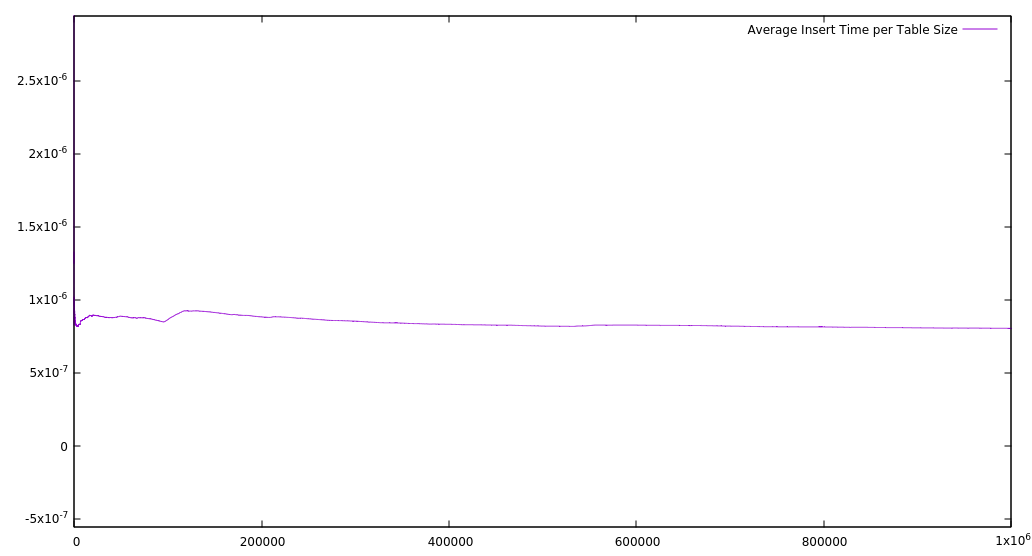This suggests that, no matter the table size, it should always take about the same time to perform an insertion, and that is a result I’m happy with. As some simple, preliminary tests, there is not much that can be inferred — perhaps a comparison between other implementations (array, linked list, etc) would be interesting. My inner skeptic also feels that running times this quick do seem a bit too good to be true, and there’s always the possibility of a bug that I haven’t noticed. I plan to design some better tests to rule out this possibility.

You can check out all the code on github. My next rough steps are supporting deletions, undo, and then working on an API. After that, multiple cursors, users — and beyond!

1. I’m using Joaqin’s estimate here

]]>
Avery Lairdalaird@cs.toronto.edu
Text Editor: Data Structures2017-09-30T00:00:00+00:002017-09-30T00:00:00+00:00https://averylaird.com/programming/the%20text%20editor/2017/09/30/the-piece-tableThe first step in building my text editor is to implement the core API. If you’re wondering why I want to do this, the original article is here.

I researched several data types, and I tried to be language agnostic. I wanted my decision to not be influenced by any particular language, and first see if there was a “best way” out there, solely based on operations. Of course, a “best way” rarely exists. However, in the case of text manipulation and storage, there are some clear “worst ways” and “better ways.”

## The Worst Way

The worst way to store and manipulate text is to use an array. Firstly, the entire file must be loaded into the array first, which raises issues with time and memory. Even worse still, every insertion and deletion requires each element in the array to be moved. There are more downsides, but already this method is clearly not practical. The array can be dismissed as an option rather quickly.

By the way: this isn’t a challenge. Please don’t try to find worse ways to manipulate text.

## A Good Way

Another option is a binary tree structure called a rope. Skip to the next section if binary trees aren’t your thing.

If you are unfamiliar with binary trees, check out this as a starting point.

Basically, the string is split into sections, and stored in the leaves. The weight of each leaf is the length of the string segment. The weight of each non-leaf node is the total length of the strings on its left subtree. For example, in the diagram1 below, node $$E$$ is a leaf with a string segment $$6$$ characters long. Therefore, it has a weight of $$6$$, as well as its parent node. However, node $$B$$ has a weight of $$9$$, because nodes $$E$$ and $$F$$ together have a length of $$9$$.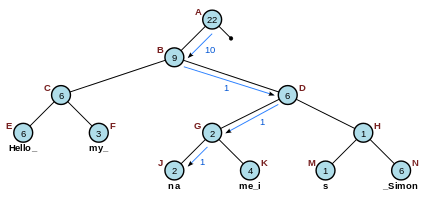This is a lot more efficient than an array. A rope has two main operations, Split and Concat (concatenation). Split splits one string into two strings at a given index, and Concat concatenates two strings into one. You can preform either an insert or delete with either of these two basic operations. To insert characters, you can split the string once (where you want to insert the content) and concatenate it twice (on either side of the inserted content). Deletions work similarly, by splitting the string twice, and concatenating them again without including the deleted content.

There’s a big downside. Using a rope is quite confusing and complicated. It’s difficult to explain even in an abstract manner. Working out the kinks in real life, while still making the code maintainable and readable, seems like a nightmare. What’s more, it still uses a lot of space. It didn’t seem like the best option yet, so I kept looking.

## A Better Way

The Gap Buffer is much simpler than the rope. The idea is this: operations on text are often localized. Most of the time, we’re not jumping all over the document. So, we create a “gap” between characters stored in an array. We keep track of how large the gap is by using pointers or array indices. Let’s examine two cases (using pointers):

Insertion
The gap is a certain size to begin with. We copy over the inserted content, and if it exceeds the size of the gap, we expand the gap.
Deletion
We shift the pointers of the gap to include the deleted content.

This is makes a lot of sense. We are plagued somewhat by the same issues as an array; under certain circumstances, if we move too far from the gap, every element in the array will have to be moved. However, it’s most likely that this is a rare occurrence for the average user. It is quite possible that the speed gained with most operations will outweigh the inefficiency of certain edge cases. In fact, the editor I’m writing this in – Emacs – uses a gap buffer, and it’s probably the fastest editor I’ve ever used. That fact alone is a pretty convincing argument to use a gap buffer. But if I’m starting from scratch, I want every aspect of the software to be the best option there is. And maybe there’s a better(est) way.

## The Better(est) Way

A couple months ago, my Dad asked me for help with a problem. He was converting one of his books to markdown, and there was an issue with the footnotes. In markdown, footnotes do not automatically number themselves2; they need to be labelled with either a number or some text, like this: [^1] or [^footnote]. The definition is the same, with a colon at the end.

He had used pandoc to mostly convert the document, but every footnote had the format [^#]. It was my job to make a script to replace every # with a number, starting from $$1$$.

Easy, right?

Well, that’s what I thought. I whipped up a regex, scanned through the document, and replaced all occurrences of the pattern with an increasing integer. And, it spat out garbage.

Why? Because I had made a really, really obvious mistake. The counter doesn’t always take up the same amount of space. The script kept overwriting content, and the offset grew larger the more footnotes were replaced. There’s a simple fix: keep track of how much more space you take up, and add that to your current position in the document. I made that one simple change, and everything worked perfectly. Without knowing it at the time, I had used a Piece Table.

The Wikipedia page for the Piece Table is only 8 lines long (Yikes!) Even more concerning, it mentions Microsoft Word among the examples of editors that use piece tables. However, the piece table is a very promising structure. What’s more, at its conception Word was lightning fast with infinite redo/undo, as explained in this interesting article by a Microsoft developer. If you have the time, it’s a cool read.

In 1998, Charles Crowley wrote a paper investigating the pros and cons of various data structures used in text editors. His paper includes the structures we covered, like gap buffers, arrays, and ropes. He concluded that – from a basis of speed, simplicity, and structure – the piece table was the leading method. From my point of view, the piece table is also the most elegant solution.

We need two buffers: the original file (read-only), and a new file that will contain all of our added text (append-only). Lastly, we have a table that has three columns: file, start, length. This is which file to use (original or new), where the text segment starts in each file (pre-edit), and the length of the segment. Here’s an example:

Original File: A_large_span_of_text   (underscores denote spaces)
New File:      English_

File           Start         Length
-----------------------------------
Original           0              2
Original           8              8
New                0              8
Original          16              4

Sequence: A_span_of_English_text


Keep in mind that the Start index is not relative to previous edits. This is something that gets handled at runtime by adding the length of each previous edit (like what I did to fix my footnote script). Since the length is already included in the table, this is a trivial step.

I like this solution the most because:

1. It’s elegant. Only the minimal amount of information is recorded.
2. It’s simple. We run through the table, and do the necessary operations. We don’t need to re-balance or traverse a binary tree.
3. It’s fast. This solution has the potential to be lightning fast. There are some additional optimizations to take into account, which are explained in detail here

The piece table method certainly has its complications, and there are different variations in implementation. It is certainly a daunting task. I’m going to see how far I get. Another article will accompany my attempts to implement the piece table method.

1. From Wikipedia

2. They should! Why aren’t they!?!?! Somebody needs to make that a markdown extension. Every time you want to insert an indexed footnote, you type [^#]. Then, it takes every footnote definition with that format and matches them up. If there’s a mismatch (like a named reference), you wonder why some of your footnotes are missing and fix it. I had to change all of my footnotes just to insert this footnote. It’s crazy.

]]>
A Text Editor2017-09-22T00:00:00+00:002017-09-22T00:00:00+00:00https://averylaird.com/programming/2017/09/22/the-text-editorLately I’ve been playing around with the idea of creating a text editor. Here’s a very quick and basic overview of what I think it should contain. The text editor has extensions, managers, projects, and a core API.

## The Editor has Extensions

For example, a user wants the editor (TE) to integrate with pandoc in some way. Or, they may want a spell checker. The user could write an extension, which behaves as additional behaviour of the API. It expands the types of calls that can be made to the API.

## The Editor has Managers

The core API makes no assumptions about how the user would like to display their content. A manager must be written for every display method (the web, desktop, etc).

## The Editor has Projects

Projects are introduced as an abstract type: a project may be anything. For example, in the case of a Jekyll project, there is a root directory (the website), some configuration information, certain commands that must be run to generate and manage the site, and so on. These can be defined in a Project, eg:

A project can be less complicated. They can be an analogue to major modes in emacs; there may be a markdown project. In this case, the markdown extension might be loaded, and the pandoc extension could be available to convert from markdown to PDF.

## The Editor has an API

The API must supply certain basic functionality. A buffer can be created, edited, and saved. Furthermore, the buffer is available to extensions. Managers and Projects have no direct access to the buffer, they must query the API.

## How is The Editor different from Emacs?

I feel The Editor fills a role Emacs does not. Extensible with Python rather than Elisp, project management (multiple directories) with different major modes, more web-friendly API. Emacs of course has all of these things in theory, but not without a lot of customization that I wish to mitigate.

## How is The Editor different from Atom?

I haven’t used atom in some time. However, I ran into similar problems of extensibility and nodejs, and project management.

## You’re Wrong; My Favourite Editor is Better

There is nothing wrong with Atom or Sublime, and I love Emacs. All of those editors are hugely customizable, expertly built, and loaded with features. My main motivation is not to disrupt the editor habitat. I wanted to work on a project, and this seemed like a cool thing to try out. We’ll see how it goes. Wish me luck!

]]>
Avery Lairdalaird@cs.toronto.edu
Describing Elliptical Orbits Programmatically2015-06-19T19:37:03+00:002015-06-19T19:37:03+00:00https://averylaird.com/2015/06/19/describing-elliptical-orbits-programmatically

This is a migrated post from my old website. If you see any odd formatting or other inconsistencies, please let me know.

Like most space nerds, I play Kerbal Space Program. I also read The Martian a couple months ago; it was a terrific book, and I highly recommend it. One of my favourite aspects of the book, which is also its claim to fame, is its very impressive intention to be as scientifically accurate as possible. I’ve always been interested in how KSP simulates orbits, but The Martian also got me thinking about how actual orbital maneuvers are planned, and the math involved. That’s why I decided to see if I could use math, and Python, to describe the orbits of Earth and Mars.

## The Math

This wikipedia article very helpfully breaks down the math into four distinct steps:

1. Compute the mean anomaly: $$M = nt, nP = 2\pi$$

$M = \frac{2\pi t}{P}$

Where $$n$$ is the mean motion, $$M$$ is the mean anomaly, and $$t$$ is the time since perihelion. It’s interesting to note that in the consolidated formula, we get the relationship $$\frac{t}{P}$$. Therefore, since $$2\pi$$ is constant, the term which defines $$M$$ is simply the ratio between time since perihelion and the orbital period. This means that any unit of time can be used, as long as it is used for both parameters.

2. Compute the eccentric anomaly $$E$$ by solving Kepler’s equation:

$M = E - \varepsilon\sin{E}$

Where $$\varepsilon$$ is the eccentricity of the orbit.

3. Compute the true anomaly $$\theta$$ by the equation:

$(1 - \varepsilon)\tan^2{\frac{\theta}{2}} = (1 + \varepsilon)\tan^2{\frac{E}{2}}$
4. Compute the heliocentric distance:

$r = a(1 - \varepsilon \cos{E})$

Where $$a$$ is the semi-major axis.

Next, we’ll translate each step in to code.

You can find all the code in one place at the end of this article

### Step One

First, you’ll need to import the math module, and install matplotlib. I recommend using a package manager to install matplotlib, eg sudo apt-get install python-matplotlib. As the first thing in your file, you should end up with:

For step one, we need to compute the mean anomaly. We need time since perihelion and the orbital period, so we’ll make those parameters:

### Step Two

In step two, we’re solving for the eccentric anomaly, and we need the mean anomaly and eccentricity to do it.

Since Kepler’s equation is transcendental, and cannot be solved algebraically, the solution has to be found numerically.

We’ve got two lines, one horizontal, one slanted with slope = 1, and the point where they intersect is our solution. Or, you could move the $$M$$ over to get $$M - E + \varepsilon\sin{E} = 0$$ and say the root is your solution. I choose the former. It looks something like this: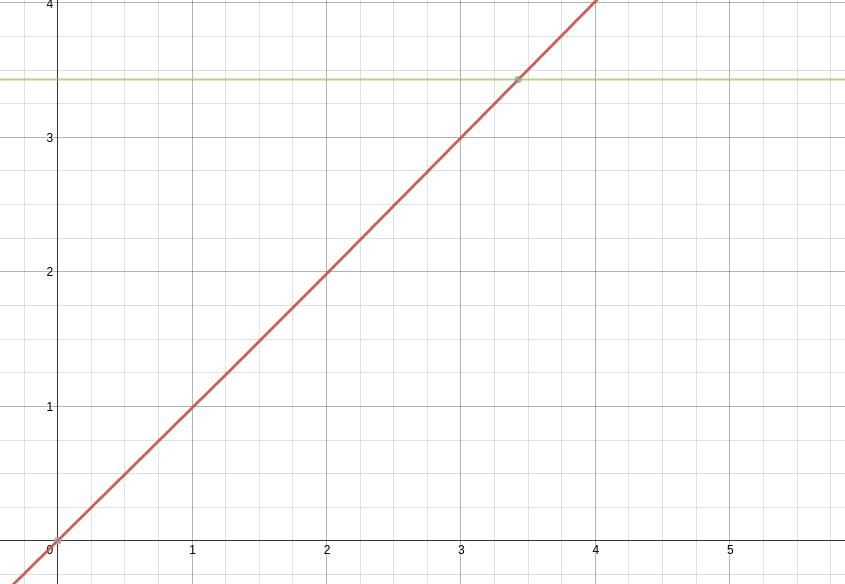We know that $$M$$, a constant value, will always be greater than $$E - \varepsilon\sin{E}$$ when $$E = 0$$. In fact, the right side of the equation is basically a slanted $$\sin{x}$$ graph. You could also think of it as a $$y = x$$ graph being sinusoidally translated up and down, where the amplitude of translation is the eccentricity of the orbit.

Knowing that the right side of the equation will always start out as being less than the left, to find the intersection point we can just increase values of $$E$$ (starting with $$E = 0$$) until the right side is equal to the left. However: we’ll be computing thousands of positions, and we want to be able to find the solution very quickly, but also with lots of precision. That’s why our algorithm should be as follows:

Initial Conditions: $$E = 0$$

• Is right side $$<$$ left side?
• Yes: increment $$E$$ by $$1$$
• No: Is right side > left side?
• Yes: decrement $$E$$ by $$0.00001$$
• No: stop

This method is fast, but can also be arbitrarily precise by adding as many decimal points to the decrement step as need.

### Step Three

This is, by far, the hardest step to implement. It’s easy to get tripped up because it involves a $$\tan$$ equation which has two solutions in a given cycle. This step will require a bit of high school trigonometry.

First of all, the right side of the equation, $$(1 + \varepsilon)\tan^2{\frac{E}{2}}$$, is a number – all of the variables are known – so let’s forget about it for now.

Normally, $$\tan$$ has a period of $$\pi$$. When you square it, the period doesn’t change, but when you divide the variable $$\theta$$ by 2, $$\tan^2{\frac{\theta}{2}}$$, then the period becomes $$2\pi$$: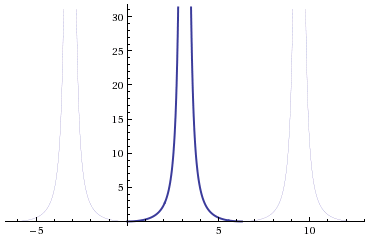Now the right side of our equation, which is a number (not a variable), will be a horizontal line which intersects with the $$\tan^2{\frac{\theta}{2}}$$ twice, like this:Now the tricky part is that we need both of those solutions. One of the solutions is for when $$0 \le t \le \frac{P}{2}$$, and the other is for when $$t \gt \frac{P}{2}$$. In other words, without the second solution, you won’t be able to calculate true anomalies for times greater than half the orbital period. My solution is this:

As you can see, this step is very similar in principle to step two, with some key differences. First, I increment by 0.1 and not 1. This is because the angles are very small to begin with, and so incrementing by 0.1 many times is much faster than decrementing by 0.00001 many times. Second, this step returns a list and not a number. The list contains both solutions. In order to get the second solution, a bit of reasoning is need.

First, the $$\tan^2{\frac{\theta}{2}}$$ graph is symmetrical in its cycle, meaning the values after $$\pi$$ can be described as a reflection of the previous values ($$0 \lt x \lt \pi$$) over a line $$x = \pi$$. This means that the distance from the first solution $$\theta_1$$ to $$\pi$$, which is $$\pi - \theta_1$$, is equal to the distance from the second solution $$\theta_2$$ to $$\pi$$. Therefore, the distance between solutions is $$2(\pi - \theta_1)$$. The value of $$\theta_2$$ can then be found by the equation:

$\theta_2 = 2(\pi - \theta_1) + \theta_1$

The solution to use will be determined later, since $$t$$ and $$P$$ are required.

Okay, we made it through the toughest part! It’s all smooth sailing from here.

### Step Four

This is where we calculate the heliocentric distance, or the planet’s distance from the sun. It is given by the equation $$r = a(1 - \varepsilon\cos{E})$$. We will need the semi-major axis, eccentricity, and eccentric anomaly as parameters:

### Tying it all together

At this point, you have all the tools you need to predict the position of a planetary body as a function of time. However, I would recommend creating one last function that handles the order of calculations and determines which true anomaly solution to use. I just made a simple, barebones one:

Lastly, I like to plot things, and I want to plot orbits. At the beginning of this article I said I wanted to predict the orbits of Earth and Mars, so here’s some example code to accomplish this:

This gives me a pretty graph that looks like this: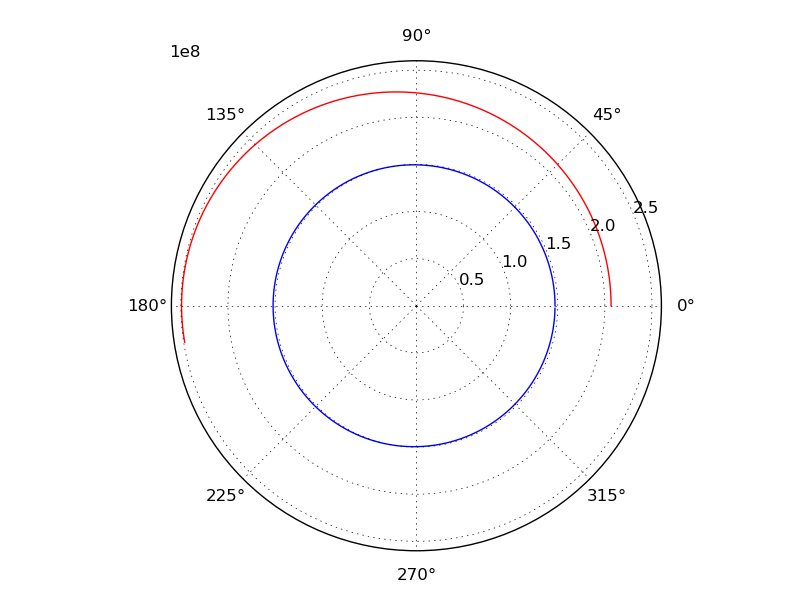## Conclusion

With this code, I see many interesting avenues of research. I can plot more planets, graph their velocity, relative distances, relative angles, etc. Eventually I plan to refine this code for actual calendar dates, and use it to determine launch dates and windows.

### Improvements

Some possible improvements could be made on the true anomaly function. Since orbits are quite often plotted one way, and sequentially, it could be optimized for this purpose by accepting the previously calculated angle as a starting point for calculating the next, instead of starting from 0 for all angles. This would be easily done with a parameter which defaults to 0.

The orbits could also be more precise by taking the gravitational influence of other bodies in to account. Additionally, when calculating relative distances more precision could be gained by taking into account the third dimension. This would require additional orbital elements, namely the orbital inclination.

### The whole !#

Here’s all the code, for those who are lazy:

## Final Thoughts

If you made it all the way to the end, I’m very impressed. Thanks for reading!

I try to be as accurate as possible, but if you see any mistakes or have any questions, comment below or feel free to email me.

]]>
Avery Lairdalaird@cs.toronto.edu